## GATE 2021 CS-Set-1

 Question 1
Consider the following sentences: (i) Everybody in the class is prepared for the exam. (ii) Babu invited Danish to his home because he enjoys playing chess. Which of the following is the CORRECT observation about the above two sentences?
 A (i) is grammatically incorrect and (ii) is ambiguous B (i) is grammatically correct and (ii) is ambiguous C (i) is grammatically correct and (ii) is unambiguous D (i) is grammatically incorrect and (ii) is unambiguous
Aptitude       Verbal
Question 1 Explanation:
i) Everybody in the class is prepared for the exam This is grammatically correct statement
ii). Babu invited Danish to his home because he enjoys playing chess.
This statement is ambiguous as there is no guarantee that Danish knows playing chess.
 Question 2

 Items Cost (₹) Profit % Marked Price (₹) P 5,400 --- 5,860 Q --- 25 10,000
Details of prices of two items P and Q are presented in the above table. The ratio of cost of item P to cost of item Q is 3:4. Discount is calculated as the difference between the marked price and  the selling price. The profit percentage is calculated as the ratio of the difference between selling price and cost, to the cost Profit %=Selling price-CostCost100.The discount on item Q, as a percentage of its marked price, is ______.
 A 25 B 5 C 10 D 12.5
Aptitude       Numerical
Question 2 Explanation:Question 3
The ratio of boys to girls in a class is 7 to 3. Among the options below, an acceptable value for the total number of students in the class is:
 A 37 B 73 C 21 D 50
Aptitude       Numerical
Question 3 Explanation:
The Ratio of boys to girls is 7:3
Number of boys = 7x
Number of girls = 3x
Total number of students = 10x
So the possible value should multiple by 10.
 Question 4
A polygon is convex if, for every pair of points, P and Q belonging to the polygon, the line segment PQ lies completely inside or on the polygon. Which one of the following is NOT a convex polygon?
 ABCDAptitude       Numerical
Question 4 Explanation:Question 5A circular sheet of paper is folded along the lines in the directions shown. The paper, after being punched in the final folded state as shown and unfolded in the reverse order of folding, will look like _______.
 ABCDAptitude       Numerical
Question 5 Explanation:Question 6
We have 2 rectangular sheets of paper, M and N, of dimensions 6 cm × 1 cm each. Sheet M is rolled to form an open cylinder by bringing the short edges of the sheet together. Sheet N is cut into equal square patches and assembled to form the largest possible closed cube. Assuming the ends of the cylinder are closed, the ratio of the volume of the cylinder to that of the cube is ______.
 A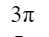BCDAptitude       Numerical
Question 6 Explanation:Question 7
______ is to surgery as writer is to ______ Which one of the following options maintains a similar logical relation in the above sentence?
 A Hospital, library B Medicine, grammar C Plan, outline D Doctor, book
Aptitude       Verbal
Question 7 Explanation:
The given statement is to fill the relationship of the subject and the action done by the subject.
Doctor is to surgery as writer is to book.
 Question 8
There are five bags each containing identical sets of ten distinct chocolates. One chocolate is picked from each bag. The probability that at least two chocolates are identical is _____
 A 0.81225 B 0.4235 C 0.6976 D 0.3024
Aptitude       Numerical
Question 8 Explanation:
Explanation :
There are 5 bags having identical sets of 10 distinct chocolates. one chocolate is drawn from each bag, probability to find at least 2 chocolate is identical
The total number of such selections possible = 10*10*10*10*10 = 10^5.
We need to select such that at least two of them are identical.
We can count the number of ways with no chocolate being repeated and subtract it from total possible ways to get the required probability.
The number of ways to select all different/distinct = 10*9*8*7*6
Required number of selections= 10^5-10*9*8*7*6 = 69760
Required probability = 69760/10^5 = 0.69760
 Question 9
Some people suggest anti-obesity measures (AOM) such as displaying calorie information in restaurant menus. Such measures sidestep addressing the core problems that cause obesity: poverty and income inequality. Which one of the following statements summarizes the passage?
 A AOM are addressing the problem superficially. B The proposed AOM addresses the core problems that cause obesity. C AOM are addressing the core problems and are likely to succeed. D If obesity reduces, poverty will naturally reduce, since obesity causes poverty.
Aptitude       Verbal
Question 9 Explanation:
The problems mentioned are poverty and income inequality. But AOMs are not addressing the main problems directly. But it is a kind of generic step taken to address obesity. So AOMs are only addressing the obesity problem superficially but not addressing the real problems.
 Question 10
Given below are two statements 1 and 2, and two conclusions I and II. Statement I: All bacteria are microorganisms. Statement II: All pathogens are microorganisms. Conclusion I: Some pathogens are bacteria. Conclusion II: All pathogens are not bacteria. Based on the above statements and conclusions, which one of the following options is logically CORRECT?
 A Only conclusion I is correct B Only conclusion II is correct C Neither conclusion I nor II is correct D Either conclusion I or II is correct
Aptitude       Verbal
 Question 11
Consider the following ANSI C function:
int SimpleFunction (int Y[], int n, int x)
{
int total = Y, loopIndex;
for (loopIndex = 1; loopIndex <= n - 1; loopIndex++)
total = x * total + Y[loopIndex]
}
Let Z be an array of 10 elements with Z[i]=1, for all i such that 0 ≤ i ≤ 9. The value returned by SimpleFunction(Z, 10, 2) is _______
 A 1023
Programming       Functions
Question 11 Explanation:
Array Z consists of 10 elements and that element's values are 1.
n=10,x=2
Initial total value is 1 => total=1.
For loop will execute 9 times.
loopindex=1, 1<=9 condition is true then
total = x * total + Y[loopIndex]= 2*1+Y=2+1=3
loopindex=2, 2<=9 condition is true then
total=2*3+Y=6+1=7
loopindex=3, 3<=9 condition is true then
total=2*7+Y=14+1 =15
loopindex=4, 4<=9 condition is true then
total= 2*15+Y=30+1=31
loopindex=5, 5<=9 condition is true then
total=2*31+Y=62+1=63
loopindex=6, 6<=9 condition is true then
total=2*63+Y=126+1=127
loopindex=7, 7<=9 condition is true then
Total =2*127+Y=254+1=255
loopindex=8, 8<=9 condition is true then
total=2*255+Y=510+1=511
loopindex=9, 9<=9 condition is true then
total=2*511+Y=1022+1=1023
loopindex=10, 10<=9 condition is false then
Total value is returned which is 1023.
You can also write generalized formulae 210-1=1023
 Question 12
The following relation records the age of 500 employees of a company, where empNo (indicating the employee number) is the key: empAge (empNo, age) Consider the following relational algebra expression: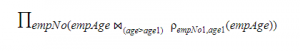What does the above expression generate?
 A Employee numbers of all employees whose age is not the maximum. B Employee numbers of only those employees whose age is the maximum. C Employee numbers of all employees whose age is not the minimum. D Employee numbers of only those employees whose age is more than the age of exactly one other employee.
Database-Management-System       Relational-Algebra
Question 12 Explanation:
The given relational algebra expression will result in employees whose age is not minimum. The given conditional join, joins the relations if the age is greater than any of the ages mentioned in the database.
 Question 13
A TCP server application is programmed to listen on port number P on host S. A TCP client is connected to the TCP server over the network. Consider that while the TCP connection was active, the server machine S crashed and rebooted. Assume that the client does not use the TCP keepalive timer. Which of the following behaviours is/are possible?
 A If the client was waiting to receive a packet, it may wait indefinitely. B If the client sends a packet after the server reboot, it will receive a RST segment. C The TCP server application on S can listen on P after reboot. D If the client sends a packet after the server reboot, it will receive a FIN segment.
Computer-Networks       TCP
Question 13 Explanation:
1. True
Since broken connections can only be detected by sending data, the receiving side will wait forever. This scenario is called a “half-open connection” because one side realizes the connection was lost but the other side believes it is still active.
2. True
The situation resolves itself when client tries to send data to server over the dead connection, and server replies with an RST packet (not FIN).
3. True
Yes, a TCP Server can listen to the same port number even after reboot.  For example, the SMTP service application usually listens on TCP port 25 for incoming requests. So, even after reboot the port 25 is assigned to SMTP.
4. False
The situation resolves itself when client tries to send data to server over the dead connection, and server replies with an RST packet (not FIN), causing client to finally to close the connection forcibly.
FIN is used to close TCP connections gracefully in each direction (normal close of connection), while TCP RST is used in a scenario where TCP connections cannot recover from errors and the connection needs to reset forcibly.
 Question 14
Consider the following C code segment:
a = b + c;
e = a + 1;
d = b + c;
f = d + 1;
g = e + f;
In a compiler, this code segment is represented internally as a directed acyclic graph (DAG). The number of nodes in the DAG is _______
 A 6
Compiler-Design       Syntax-tree-and-context-flow-graph
 Question 15
Consider the following expression.The value of the above expression (rounded to 2 decimal places) is _______
 A 0.25
Engineering-Mathematics       Calculus
Question 15 Explanation:Question 16
Consider a dynamic hashing approach for 4-bit integer keys:
1. There is a main hash table of size 4.
2. The 2 least significant bits of a key is used to index into the main hash table.
3. Initially, the main hash table entries are empty.
4. Thereafter, when more keys are hashed into it, to resolve collisions, the set of all keys corresponding to a main hash table entry is organized as a binary tree that grows on demand.
5. First, the 3rd least significant bit is used to divide the keys into left and right subtrees.
6. To resolve more collisions, each node of the binary tree is further sub-divided into left and right subtrees based on the 4th least significant bit.
7. A split is done only if it is needed, i.e., only when there is a collison.
Consider the following state of the hash table.Which of the following sequences of key insertions can cause the above state of the hash table (assume the keys are in decimal notation)?
 A 10, 9, 6, 7, 5, 13 B 9, 5, 13, 6, 10, 14 C 9, 5, 10, 6, 7, 1 D 5, 9, 4, 13, 10, 7
Data-Structures       Hashing
Question 16 Explanation:
The key insertions 10, 9, 6, 7, 5, 13 would result in the state of the given hash table.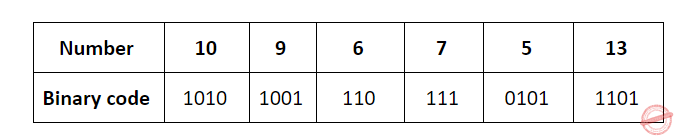The keys are inserted into the main hash table based on their 2 least significant bits.
→ The keys 5, 9, 13 will be inserted in the table with entry “01”.
→ The keys 6 and 10 will be inserted in the table with entry “10”
→ The keys 7 will be inserted in the table with entry “11”

→ Now the entry index 01 has collisions. Check the 3rd least significant bit of the keys 9,5,13. The 3rd least significant bit of the key is 0. Hence, key “9” placed as the left child of “01”. There will be collisions again in between the keys 5 and 13 which need to splitted.
Now check the 4th least significant bit of keys 5 and 13. The key 5 is placed as a left child because the 4th least significant is “0” and the key “13” is placed as right child because its 4th least significant is 1.
→ Now the entry index 10 has a collision. Check the 3rd least significant bit of the keys 10 and 6. The 3rd last significant bit of the key is 0. Hence, “10” is placed as the left child of index “10”.
The 3rd least significant value of “6” is 1. So, we are placed as the right child of index ”10”.
 Question 17
Let p and q be two propositions. Consider the following two formulae in propositional logic.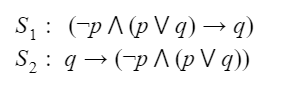Which one of the following choices is correct?
 A Both S1and S2 are tautologies. B Neither S1and S2 are tautology. C S1is not a tautology but S2is a tautology. D S1is a tautology but S2is not a tautology.
Engineering-Mathematics       Propositional-Logic
Question 17 Explanation:

A tautology is a formula which is "always true" . That is, it is true for every assignment of truth values to its simple components.

Method 1:
S1: (~p ^ (p Vq)) →q
The implication is false only for T->F condition.
Let's consider q as false, then
(~p ^ (p Vq)) will be (~p ^ (p VF)) = (~p ^ (p)) =F.
It is always F->F which is true for implication. So there are no cases that return false, thus its always True i.e. its Tautology.

S2:

q->(~p (p Vq))

The false case for implication occurs at T->F case.
Let q=T then (~p (p Vq))  = (~p (p VT))= ~p. (It can be false for p=T).
So there is a case which yields T->F = F. Thus its not Valid or Not a Tautology.

Method 2: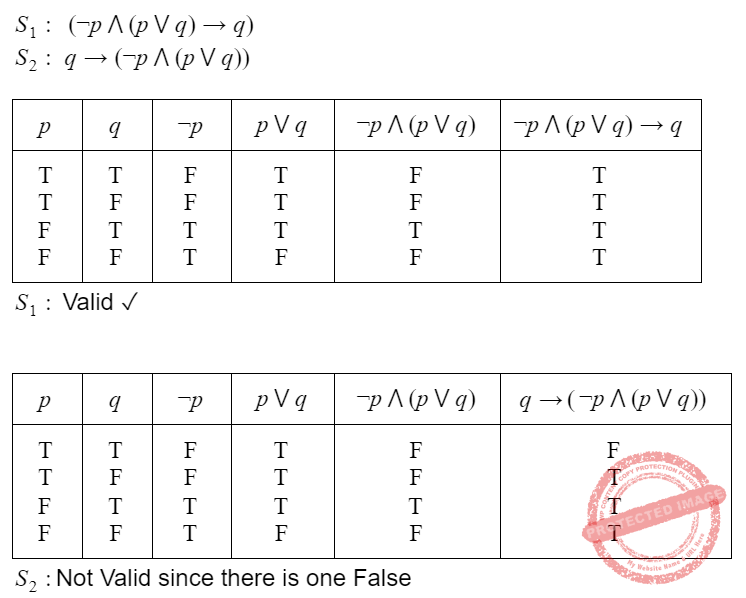Question 18
Consider the following sequence of operations on an empty stack.
push(54); push(52); pop(); push(55); push(62); s=pop();
Consider the following sequence of operations on an empty queue.
enqueue(21); enqueue(24); dequeue(); enqueue(28); enqueue(32); q = dequeue();
The value of s + q is ________
 A 86
Data-Structures       Queues-and-Stacks
Question 18 Explanation:

push(54) ⇒ 54

push(52)=> 54, 52(top)

pop() ⇒ 54 (top)

push(55)==> 54, 55(top)

push(62) ⇒ 54,55,62(top)

s=pop() ⇒ 62 will store into the variable s then s=62

enqueue(21) ⇒     (front) 21(rear)

enqueue(24) ⇒   (Front)21, 24(rear)

dequeue()==> (front) 24(rear)

enqueue(28) ===> (front) 24,28 (rear)

enqueue(32)====>(front) 24,28,32 (rear)

q=dequeue() ⇒ value 24 will store into the variable “q”

q=24

S+q =62+24 =86

 Question 19
Consider the following representation of a number in IEEE 754 single-precision floating point format with a
bias of 127.
S : 1      E : 10000001      F : 11110000000000000000000
Here S, E and F denote the sign, exponent and fraction components of the floating point representation.
The decimal value corresponding to the above representation (rounded to 2 decimal places) is _______
 A -7.75
Digital-Logic-Design       Number-Systems
Question 19 Explanation:

Sign bit S= 1. The given number is a negative number.

Biased Exponent E = 27 + 1= 129

Actual Exponent e = E-127

= 129- 127

= 2

The decimal value= (-1)s x 1.M x 2e

= (-1) 1 x 1.1111 x 22

= - (111.11)

= - (7 + 0.75)

= -7.7

 Question 20
Let the representation of a number in base 3 be 210. What is the hexadecimal representation of the number?
 A 21 B 528 C D2 D 15
Digital-Logic-Design       Number-Systems
Question 20 Explanation:

On converting (210)3 in decimal, we will get:=>

2*32+1*3=2*9+3=2110

=>(15)16

 Question 21
Let ri(z) and wi(z) denote read and write operations respectively on a data item z by a transaction Ti. Consider the following two schedules.
S1: r1(x) r1(y) r2(x) r2(y) w2(y) w1(x)
S2: r1(x) r2(x) r2(y) w2(y) r1(y) w1(x)
Which one of the following options is correct?
 A S1is not conflict serializable, and S2 is conflict serializable. B Neither S1nor S2is conflict serializable. C Both S1and S2are conflict serializable. D S1is conflict serializable, and S2is not conflict serializable.
Database-Management-System       Transactions
Question 21 Explanation:Question 22
A sender (S) transmits a signal, which can be one of the two kinds: H and L with probabilities 0.1 and 0.9 respectively, to a receiver (R). In the graph below, the weight of edge (u, v) is the probability of receiving v when u is transmitted, where u, v ∈ {H, L}. For example, the probability that the received signal is L given the transmitted signal was H, is 0.7.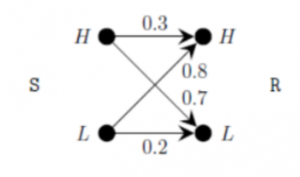If the received signal is H, the probability that the transmitted signal was H (rounded to 2 decimal places) is _______
 A 0.04
Engineering-Mathematics       Probability-and-statistics
Question 22 Explanation:

Bayes theorem:
Probability of event A happening given that event B has already happened is
P(A/B) = P(B/A)*P(A)  / P(B)

S can send signal  to H with 0.1 probability, S can send signal to L with 0.9 probability.
The complete diagram can beProbability that H Transmitted (H_t) given that H received (H_r)is

P( H_t  / H_r) = P( H_r/ H_t) * P(H_t)  / P(H_r)

It can be observed from the graph that H can receive in two ways (S to H to H) and (S to L to H)
The P(H_r) = 0.1*0.3 + 0.9*0.8= 0.03+0.72 = 0.75

P(H transmitted ) = 0.1  i.e.

P( H_t  / H_r) = P( H_r/ H_t) * P(H_t)  / P(H_r)
= 0.3*0.1 / 0.75 = 0.04

 Question 23
In an undirected connected planar graph G, there are eight vertices and five faces. The number of edges in G is ______
 A 11
Engineering-Mathematics       Graph-Theory
Question 23 Explanation:

v - e + f = 2

v is number of vertices

e is number of edges

f is number of faces including bounded and unbounded

8-e+5=2

=> 13-2 =e

The number of edges  are =11

 Question 24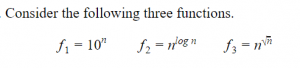Which one of the following options arranges the functions in the increasing order of asymptotic growth rate?
 A f2, f3, f1 B f3, f2, f1 C f2, f1, f3 D f1, f2, f3
Algorithms       Asymptotic-Complexity
Question 24 Explanation:

The asymptotic notation order should be

Constant < logarithmic < linear < polynomial < exponential < factorial

F2 and F3 → Polynomial

F1 → Exponential

By the order of asymptotic notations F1 is definitely larger.

Method-1:

Consider n=100

F1 : 100 ^100 ⇒ 1.e+200

F2 : N^log(100) base 2 ⇒  100 ^ log(100) base 2 ⇒ 100^6.6438561897747 = 1,93,96,00,91,15,564.181300016469223466

F3 : N^Sqrt(n) ====> 100^Sqrt(100) ⇒ 100^10 ⇒ 10,00,00,00,00,00,00,00,00,000

Method-2:

We can apply "log" on both sides.

log(F1)=nlog10 (base 2)

log(F2)=(logn)^2 = logn * logn (base 2)

log(F3)=sqrt(n)logn (base 2)

 Question 25The number of strings of length 100 accepted by the above pushdown automaton is ______
 A 50
Theory-of-Computation       Push-Down-Automata
Question 25 Explanation: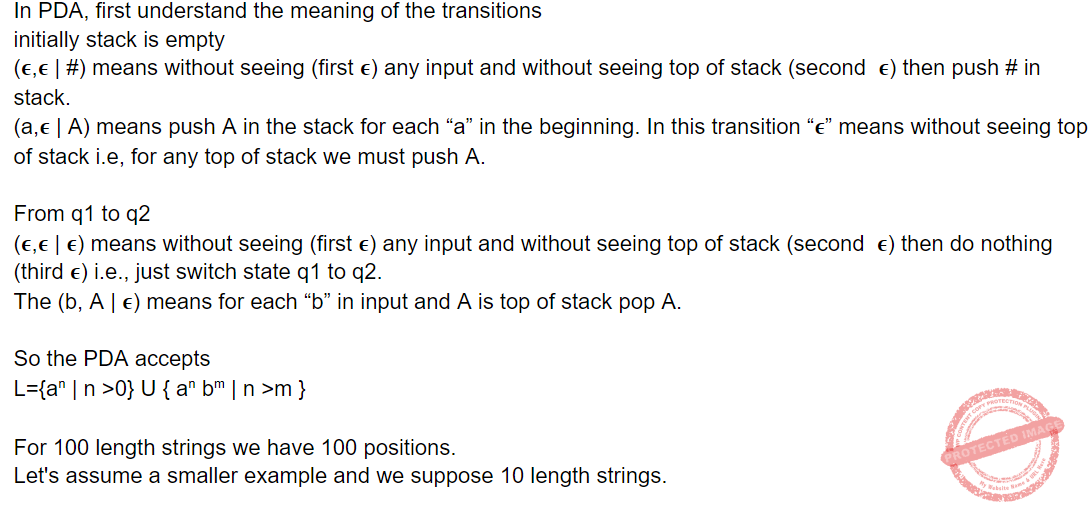Question 26
Consider the following statements.
S1:Every SLR(1) grammar is unambiguous but there are certain unambiguous grammars that  are not SLR(1).
S2: For any context-free grammar, there is a parser that takes at most O(n3 )
Which one of the following options is correct?
 A S1is true and S2is true B S1is true and S2is false C S1is false and S2is true D S1is false and S2is false
Compiler-Design       Parsers
Question 26 Explanation:

Every unambiguous grammar need not be SLR(1). As we know some unambiguous grammar which is CLR(1)  but not SLR(1).  So S1 is true.

Any CFG (which is in CNF form) can be parsed by CYK algorithm in O(n3) where n is length of string. Although it is not given that CFG is in CNF form but since we can convert any CFG in CNF form so S2 is true

 Question 27
Consider the relation R(P, Q, S, T, X, Y, Z, W) with the following functional dependencies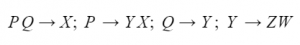Consider the decomposition of the relation R into the consistent relations according to the following two decomposition schemes.
D1: R=[(P,Q,S,T); (P,T,X); (Q,Y); (Y,Z,W)]
D2: R=[(P,Q,S);(T,X);(Q,Y);(Y,Z,W)]
Which one of the following options is correct?
 A D1is a lossy decomposition, but D2is a lossless decomposition. B Both D1and D2are lossy decompositions. C Both D1and D2are lossless decompositions. D D1is a lossless decomposition, but D2is a lossy decomposition.
Database-Management-System       Normalization
Question 27 Explanation:

Given functional dependencies set:

PQ->X

P->YX

Q->Y

Y->ZW

• While merging the tables there should be some common attribute(s) and it should be a candidate key of one of the tables.• R1 should be merged with R2 because PT is a key of R2.
• R3 should be merged with PQSTX because Q is a key of R3.
• R4 should be merged with PQSTXY because Y is a key of R4.• R1 should be merged with R3 because Q is a key of R3.
• R4 should be merged with PQSY because Y is a key of R4.
• Now, there is no common attribute in between R2(TX) and PQSYZW.
• Hence, D2 is lossy decomposition.
 Question 28
Consider the two statements.
S1: There exist random variables X and Y such that
EX-E(X)Y-E(Y)2>Var[X] Var[Y]
S2: For all random variables X and Y,
CovX,Y=E|X-E[X]| |Y-E[Y]|
Which one of the following choices is correct?
 A S1is false, but S2is true. B Both S1and S2are true. C S1is true, but S2is false. D Both S1and S2 are false.
Engineering-Mathematics       Probability-and-statistics
Question 28 Explanation:
Variance(X) = Var[X]= E((X-E(X))^2)
For a dataset with single values, we have variance 0. EX-E(X)Y-E(Y)2>Var[X] Var[Y]
This leads to inequance of 0>0 which is incorrect.Its not |x-E(x)|. Thus S2 is also incorrect.
 Question 29
Define Rn< >to be the maximum amount earned by cutting a rod of length n metres into one or more pieces of integer length and selling them. For i > 0, let p[i] denote the selling price of a rod whose length is i meters. Consider the array of prices:
p = 1, p = 5, p = 8, p = 9, p = 10, p = 17, p = 18
Which of the following statements is/are correct about R7?
 A R7is achieved by three different solutions. B R7=18 C R7=19 D R7cannot be achieved by a solution consisting of three pieces.
Algorithms       Dynamic-Programming
Question 29 Explanation:
The rod length of R7 is 18.There are 3 different possible ways to get the maximum amount.
P + P → 17+1 = 18
P + P + P → 5+5+8 = 18
P → 18 = 18
 Question 30
An articulation point in a connected graph is a vertex such that removing the vertex and its incident edges disconnects the graph into two or more connected components. Let T be a DFS tree obtained by doing DFS in a connected undirected graph G. Which of the following options is/are correct?
1. Root of T can never be an articulation point in G.
2. If u is an articulation point in G such that x is an ancestor of u in T and y is a descendent of u in T, then all paths from x to y in G must pass through u.
3. A leaf of T can be an articulation point in G.
4. Root of T is an articulation point in G if and only if it has 2 or more children
 A 4
Data-Structures       BFS-and-DFS
Question 30 Explanation:
Statement-1: FALSE: Root of T can never be an articulation point in G.
Statement-2:
Example-1:
If u is an articulation point in G such that x is an ancestor of u in T and y is a descendent of u in T, then all paths from x to y in G must pass through u.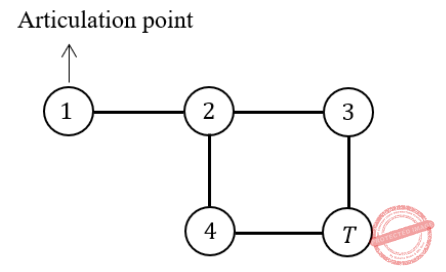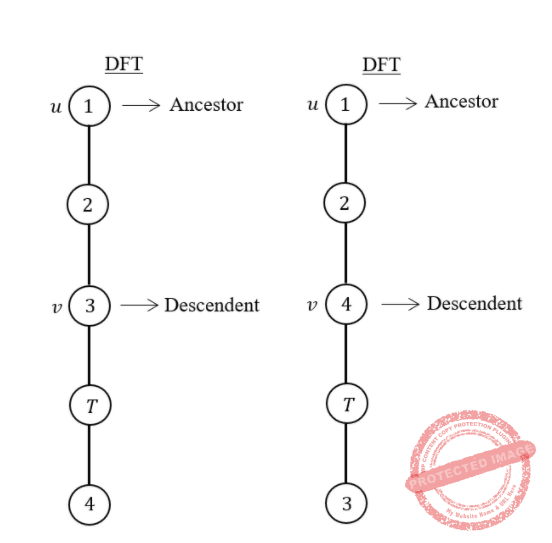Here 2 and 6 are articulation points. If you consider node-1 ancestor and node-3 descendent, then without passing through from node -2, there exists a path from one node to another node.
Path from node-1 to node-3 If you consider node-5 ancestor and node-4 descendent, then without passing through from node-6, there exists a path from one node to another node.
Path from node-4 to node-5
The given statement is not TRUE for all cases. So, the given statement is FALSE.
Statement-3: FALSE: Leafs of a DFS-tree are never articulation points.
Statement-4: TRUE: The root of a DFS-tree is an articulation point if and only if it has at least two children.Node 2 is an AP because any node from the first subtree (1, 2) is connected to any node from the second subtree (4, 5, 6, 7, 8) by a path that includes node 2. If node 2 is removed, the 2 subtrees are disconnected.
 Question 31
In the context of operating systems, which of the following statements is/are correct with respect to paging?
 A Page size has no impact on internal fragmentation. B Multilevel paging is necessary to support pages of different sizes. C Paging incurs memory overheads. D Paging helps solve the issue of external fragmentation.
Operating-Systems       Memory-Management
Question 31 Explanation:
1. False, Large page size may lead to higher internal fragmentation.
2. False, To support pages of different sizes, the Instruction set architecture should support it. Multi-level paging is not necessary.
3. True, The page table has to be stored in main memory, which is an overhead.
4. True, Paging avoids the external fragmentation.
 Question 32
Three processes arrive at time zero with CPU bursts of 16, 20 and 10 milliseconds. If the scheduler has prior knowledge about the length of the CPU bursts, the minimum achievable average waiting time for these three processes in a non-preemptive scheduler (rounded to nearest integer) is _________ milliseconds.
 A 12
Operating-Systems       CPU-Scheduling
Question 32 Explanation:
Minimum average waiting time amongst non-preemptive scheduling is given by SJF. So, avg waiting time = (0+10+26)/3 = 12 ms.
 Question 33
Consider the following instruction sequence where registers R1, R2 and R3 are general purpose and MEMORY[X] denotes the content at the memory location X.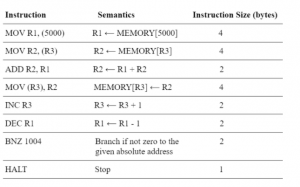Assume that the content of the memory location 5000 is 10, and the content of the register R3 is 3000. The content of each of the memory locations from 3000 to 3010 is 50. The instruction sequence starts from the memory location 1000. All the numbers are in decimal format. Assume that the memory is byte addressable.
After the execution of the program, the content of memory location 3010 is _______
 A 50
Computer-Organization       Machine-Instructions
Question 33 Explanation:

Register R1 will get value 10 from location 5000. So the loop in the given program will run for 10 times as the loop condition is based on ‘Dec R1’. When R1 is 0 then the loop terminates.

In these 10 iterations of the loop contents of memory locations 3000 to 3009 are changed as initial value of R3 is 3000 and in each iteration we update M[R3] ← R2 and the value of R3 is incremented to the next location 3001, 3002 etc.

But the value in location 3010 is unchanged and it will be the same as the initial value which is 50.

 Question 34
Consider the sliding window flow-control protocol operating between a sender and a receiver over a full-duplex error-free link. Assume the following:
• The time taken for processing the data frame by the receiver is negligible.
• The time taken for processing the acknowledgement frame by the sender is negligible.
• The sender has an infinite number of frames available for transmission.
• The size of the data frame is 2,000 bits and the size of the acknowledgment frame is 10 bits.
• The link data rate in each direction is 1 Mbps (=106bits per second).
• One way propagation delay of the link is 100 milliseconds.
The minimum value of the sender’s window size in terms of the number of frames, (rounded to the nearest integer) needed to achieve a link utilization of 50% is ______.
 A 51
Computer-Networks       Sliding-Window-Protocol
Question 34 Explanation:

Tt(packet) = L / B.W => 2000 bits / 10^6 bps = 2  x 10^-3 sec = 2 millisec

Tt(Ack) = L / B.W. => 10 bits / 10^6 bps = 10^-5 sec = 10^-2 millisec = 0.01 millisec

Tp = 100 millisec

Total time = Tt(packet) + 2 x Tp + Tt(Ack)

=> 2 + 2 x 100 + 0.01 = 202.01 millisec

Efficiency = 50 % = ½

Efficiency = Useful Time /  Total time

½ = n x Tt / Total time

=> 2 x n x Tt =  Total time

=>2 x n x 2 = 202.01

=> n = 202.01 / 4 => 50.50

For minimum, we have to take ceil, Hence size of window = 51

 Question 35
For a Turing machine M, <M> denotes an encoding of M. Consider the following two languages.
L1=〈M〉|M takes more than 2021 steps on all inputs
L2=〈M〉|M takes more than 2021 steps on some input
Which one of the following options is correct?
 A Both L1and L2 are undecidable. B L1is undecidable and L2is decidable. C L1is decidable and L2is undecidable. D Both L1and L2 are decidable.
Theory-of-Computation       Decidability-and-Undecidability
Question 35 Explanation:

L1 is decidable.

We can take all strings of length zero to length 2021.

If TM takes more than 2021 steps on above inputs then definitely it will take more than 2021 steps on all input greater than length 2021.

If TM takes less than 2021 steps:

In such a case suppose TM  takes less than 2021 steps (let's say  2020 steps ) for string length 2021, 2022, 2023, then definitely TM  will take 2020 steps for all input greater than 2023. Hence in both cases it is decidable.

Similarly L2 is also decidable. If we can decide for all inputs then we can decide for some inputs also.

 Question 36
Consider the following statements.
S1:The sequence of procedure calls corresponds to a preorder traversal of the activation tree.
S2:The sequence of procedure returns corresponds to a postorder traversal of the activation tree.
Which one of the following options is correct?
 A S1is false and S2is false B S1is true and S2is true C S1is true and S2is false D S1is false and S2is true
Data-Structures       Graphs-and-Tree
Question 36 Explanation:

The given statements are true, they are well known facts.

1. The sequence of procedure calls corresponds to a preorder traversal of the activation tree.
2. The sequence of returns corresponds to a postorder traversal of the activation tree.
 Question 37
Let G be a group of order 6, and H be a subgroup of G such that 1 < |H| < 6.
Which one of the following options is correct?
 A G is always cyclic, but H may not be cyclic. B Both G and H are always cyclic. C G may not be cyclic, but H is always cyclic. D Both G and H may not be cyclic.
Engineering-Mathematics       Group-Theory
Question 37 Explanation:

If ‘G’ is a group with sides 6, its subgroups can have orders 1, 2, 3, 6.

(The subgroup order must divide the order of the group)

Given ‘H’ can be 1 to 6, but 4, 5 cannot divide ‘6’.

Then ‘H’ is not a subgroup.

G can be cyclic only if it is abelian. Thus G may or may not be cyclic.
The H can be cyclic only for the divisors of 6 and H cannot be cyclic for any non divisors of 6.

 Question 38
A relation R is said to be circular of aRb and bRc together imply cRa. Which of the following options is/are correct?
 A If a relation S is reflexive and circular, then S is an equivalence relation. B If a relation S is transitive and circular, then S is an equivalence relation. C If a relation S is circular and symmetric, then S is an equivalence relation. D If a relation S is reflexive and symmetric, then S is an equivalence relation.
Engineering-Mathematics       Set-Theory
Question 38 Explanation:

Theorem: A relation R on a set A  is an equivalence relation if and only if it is reflexive and circular.

For symmetry, assume that x, y ∈ A so that xRy, lets check for  yRx.

Since R is reflexive and y ∈ A, we know that yRy. Since R is circular and xRy and yRy, we know that yRx. Thus R is symmetric.

For transitivity, assume that x, y, z ∈ A so that xRy and yRz. Check for  xRz. Since R is circular and xRy and yRz, we know that zRx. Since we already proved that R is symmetric, zRx implies that xRz. Thus R is transitive.

 Question 39
Consider the following Boolean expression.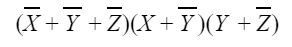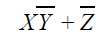ABCDDigital-Logic-Design       Boolean-Function
Question 39 Explanation:XY’+Z’ is a minimal SoP expression which represents the function (X,Y,Z).

The expression XY’ + YZ’ + X’Y’Z’ can be reduced to XY’+Z’

XY’ + YZ’ + X’Y’Z

= Y’(X+X’Z’) + YZ

= Y’(X+Z’) + Y

= XY’ + Y’Z’ + YZ’

= XY’ + (Y’+Y)Z’

= XY’ + Z’.

The expression (X+Z’)(Y’+Z’) is a PoS expression which also represents the same function (X,Y,Z).

 Question 40
Consider two hosts P and Q connected through a router R. The maximum transfer unit (MTU) value of the link between P and R is 1500 bytes, and between R and Q is 820 bytes. A TCP segment of size 1400 bytes was transferred from P to Q through R, with IP identification value as 0x1234. Assume that the IP header size is 20 bytes. Further, the packet is allowed to be fragmented, i.e., Don’t Fragment (DF) flag in the IP header is not set by P. Which of the following statements is/are correct?
 A If the second fragment is lost, R will resend the fragment with the IP identification value 0x1234. B If the second fragment is lost, P is required to resend the whole TCP segment. C Two fragments are created at R and the IP datagram size carrying the second fragment is 620 bytes. D TCP destination port can be determined by analysing only the second fragment.
Computer-Networks       IPv4-and-Fragmentation
Question 40 Explanation:Question 41A 3
Engineering-Mathematics       Linear-Algebra
Question 41 Explanation:Question 42
The lifetime of a component of a certain type is a random variable whose probability density function is exponentially distributed with parameter 2. For a randomly picked component of this type, the probability that its lifetime exceeds the expected lifetime (rounded to 2 decimal places) is _______.
 A 0.37
Engineering-Mathematics       Probability-and-statistics
Question 42 Explanation: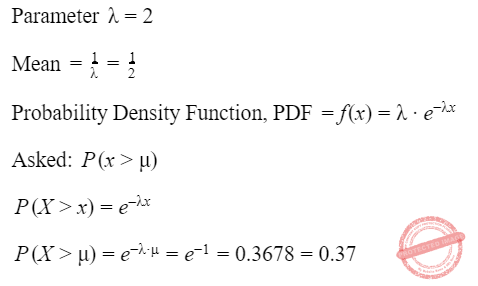Question 43
Consider the following grammar (that admits a series of declarations, followed by expressions) and the associated syntax directed translation (SDT) actions, given as pseudo-code:
P ⟶ D* E*
D ⟶ int ID {record that ID.lexeme is of type int}
D ⟶ bool ID {record that ID.lexeme is of type bool}
E ⟶ E1 + E2 {check that E1.type = E2.type = int; set E.type := int}
E ⟶ !E1 {check that E1.type = bool; set E.type := bool}
E ⟶ ID {set E.type := int}
With respect to the above grammar, which one of the following choices is correct?
 A The actions can be used to type-check syntactically correct integer variable declarations and integer expressions. B The actions will lead to an infinite loop. C The actions can be used to type-check syntactically correct boolean variable declarations and boolean expressions. D The actions can be used to correctly type-check any syntactically correct program.
Compiler-Design       Syntax-Directed-Translation
Question 43 Explanation:
This SDT will never lead to infinite loop so option 2 is false. This SDT is type checking for bool as well as integer variables, hence this SDT can be used to correctly type-check any syntactically correct program involving boolean and integer variables.
 Question 44
Suppose a database system crashes again while recovering from a previous crash. Assume checkpointing is not done by the database either during the transactions or during recovery. Which of the following statements is/are correct?
 A The same undo and redo list will be used while recovering again. B The database will become inconsistent. C The system cannot recover any further. D All the transactions that are already undone and redone will not be recovered again.
Database-Management-System       Recovery-Management-Technichque
Question 44 Explanation:
Suppose a database system crashes again while recovering it from the previous crash then also we need to make use of the redo and undo list to recover the system.
 Question 45
Which of the following standard C library functions will always invoke a system call when executed from a single-threaded process in a UNIX/linux operating system?
 A sleep B strlen C malloc D exit
Operating-Systems       System-Calls
Question 45 Explanation:
• A sleep system call takes a time value as a parameter, specifying the minimum amount of time that the process is to sleep before resuming execution. The parameter typically specifies seconds, although some operating systems provide finer resolution, such as milliseconds or microseconds.
• strlen() is not system call.
• The function call malloc() is a library function call that further uses the brk() or sbrk() system call for memory allocation
• Exit also system call
 Question 46
Consider the following two statements.
Which of the following choices is correct?
 A Both S1and S2are true. B S1is true and S2is false. C S1is false and S2is true. D Both S1and S2are false.
Computer-Networks       Network-protocols
Question 46 Explanation:
 Question 47
Consider the following pseudocode, where S is a semaphore initialized to 5 in line#2 and counter is a shared variable initialized to 0 in line#1. Assume that the increment operation in line#7 is not atomic.
1. int counter = 0
2. Semaphore S = init(5);
3. void parop(void)
4. {
5.       wait(S);
6.       wait(S);
7.       counter++;
8.       signal(S);
9.       signal(S);
10. }
If five threads execute the function parop concurrently, which of the following program behaviour(s) is/are possible?
 A The value of counter is 1 after all the threads successfully complete the execution of parop. B The value of counter is 5 after all the threads successfully complete the execution of parop. C The value of counter is 0 after all the threads successfully complete the execution of parop. D There is a deadlock involving all the threads.
Operating-Systems       Semaphores
Question 47 Explanation:

count =0

S=5

func()

{

wait();

wait();

count++;

signal();

signal();

}

No. of resources >= No. of process (Req-1) + 1

Here, no. of resources = 5 (semaphore value)

Each thread requires = 2 resources (wait call)

5 ≥ 5* (2-1)+1

≱ 6. So deadlock is possible.

This occurs when all 5 threads get blocked on first wait().

(2) count =5 is possible

When all threads enter CS and execute count++ sequentially.

(3) Count=1 is possible.

Assembly level:

inc R0, 1

write count, R0MC R0, 1, so R0=1

Write R0 to count.

So, Count=1.

 Question 48
Consider the following context-free grammar where the set of terminals is {a, b, c, d, f}Which one of the following choices represents the correct combination for the numbered cells in the parsing table (“blank” denotes that the corresponding cell is empty)?
 A ① S ⟶ Rf ② S ⟶ Rf ③ T ⟶ ∊ ④ T ⟶ ∊ B ① blank ② S ⟶ Rf ③ T ⟶ ∊ ④ T ⟶ ∊ C ① S ⟶ Rf ② blank ③ blank ④ T ⟶ ∊ D ① blank ② S ⟶ Rf ③ blank ④ blank
Compiler-Design       Parsers
Question 48 Explanation:Question 49
Consider the following language.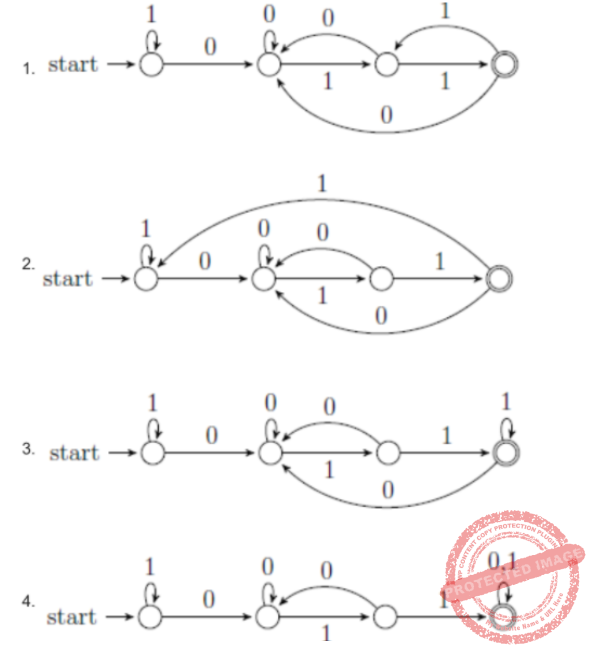A 2
Theory-of-Computation       DFA
Question 49 Explanation:

Option A accepts string “01111” which does not end with 011 hence wrong.

Option C accepts string “0111” which does not end with 011 hence wrong.

Option D accepts string “0110” which does not end with 011 hence wrong.

Option B is correct.

The NFA for language in which all strings ends with “011”

[Image_Link]">
 Question 50
A five-stage pipeline has stage delays of 150, 120, 150, 160 and 140 nanoseconds. The registers that are used between the pipeline stages have a delay of 5 nanoseconds each. The total time to execute 100 independent instructions on this pipeline, assuming there are no pipeline stalls, is ______ nanoseconds.
 A 17160
Computer-Organization       Pipelining
Question 50 Explanation:

In a pipeline with k-stages, number of cycles to execute n instructions = (k+n-1) cycles

Here k = 5, n = 100

So we need a total of 5+100-1 = 104 cycles.

Clock cycle time = maximum of all stage delays + register delay

= max(150, 120, 150, 160, 140) + 5 = 160+5 = 165 ns

Time in ns = 104*165 = 17160ns

 Question 51
Consider a computer system with a byte-addressable primary memory of size 232bytes. Assume the computer system has a direct-mapped cache of size 32 KB (1 KB = 210bytes), and each cache block is of size 64 bytes. The size of the tag field is ______ bits.
 A 17
Computer-Organization       Cache
Question 51 Explanation:

Given that the main memory is 2^32 bytes. So the physical address is 32 bits long.

Since it is a direct mapped cache the physical address is divided into  fields as (TAG, LINE, OFFSET).

Cache size is 32KB = 2^15 Bytes.

Block size is 64 bytes = 2^6 bytes, so OFFSET needs 6 bits.

Number of blocks in the cache = 2^15//2^6 = 2^9, So LINE number needs 9 bits.

TAG bits = 32 - 9 - 6 = 17 bits.

 Question 52
Let G = (V, E) be an undirected unweighted connected graph. The diameter of G is defined as:Let M be the adjacency matrix of G.
Define graph G2on the same set of vertices with adjacency matrix N, whereWhich one of the following statements is true?
 ABCDEngineering-Mathematics       Graph-Theory
Question 52 Explanation:Question 53
Consider the following undirected graph with edge weights as shown:The number of minimum-weight spanning trees of the graph is _______
 A 3
Algorithms       Minimum-Spanning-Tree
Question 53 Explanation:

To find the number of spanning trees using 2 methods:

1. If graph is complete, use n^n-2 formula
2. If graph is not complete, then use kirchhoff theorem.

Steps in Kirchoff’s Approach:

(ii) Replace all non-diagonal is by – 1.

(iii) Replace all diagonal zero’s by the degree of the corresponding vertex.

(iv) Co-factors of any element will give the number of spanning trees.

Using the Kirchhoff theorem will take lot of time because the number of vertices are 9.

So, we use brute force technique to solve the problem with the help of Kruskal's algorithm.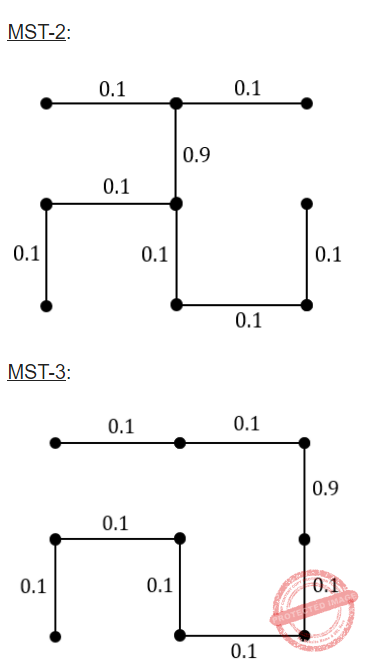Question 54
Let <M> denote an encoding of an automaton M. Suppose that Σ = {0,1}. Which of the following languages is/are NOT recursive?
 A L = { | M is a DFA such that L(M) = Σ*} B L = { | M is a DFA such that L(M) = ∅} C L = { | M is a PDA such that L(M) = Σ*} D L = { | M is a PDA such that L(M) = ∅}
Theory-of-Computation       Recursive-and-Recursively-Enumerable-Language
Question 54 Explanation:Question 55
Consider a linear list based directory implementation in a file system. Each directory is a list of nodes, where each node contains the file name along with the file metadata, such as the list of pointers to the data blocks.Consider a given directory foo. Which of the following operations will necessarily require a full scan of foo for successful completion?
 A Deletion of an existing file from foo B Opening of an existing file in foo C Creation of a new file in foo D Renaming of an existing file in foo
Operating-Systems       File-system
Question 55 Explanation:
Renaming and creating a new file requires us to search the complete inode for duplicate names (to check if the same name already exists). There is no such problem in deleting and opening a file, as duplicate names are not possible while they are created.
 Question 56
Consider the following recurrence relation.Which one of the following options is correct?
 ABCDAlgorithms       Time-Complexity
Question 56 Explanation:Question 57
There are 6 jobs with distinct difficulty levels, and 3 computers with distinct processing speeds. Each job is assigned to a computer such that:
• The fastest computer gets the toughest job and the slowest computer gets the easiest job.
• Every computer gets at least one job.
The number of ways in which this can be done is ______
 A 65
Operating-Systems       CPU-Scheduling
Question 57 Explanation:

Let the levels be  1,2,3,4,5,6. (1 is the least difficult, 6 is the most difficult level)
Let the computers be F,M,S( fast, medium, slow).

As per the given constraint,  1 must be given to F and 6 must be given to S.
Now we are left with 2,3,4,5 and  F being assigned 1, S being assigned 6 and M being assigned none.
Another constraint is that, every computer must be assigned atleast one.
So compute with assigning one job to M, two jobs to M, three jobs to M and four jobs to M.
Assigning one job to M: we can assign 1 out of 4 jobs in (4C1 ways) and remaining 3 jobs to F,S in  2*2*2 = 8  ways. (each job has two options, either F or S),

Assigning two jobs to M: we can select two jobs from 4 in 4C2 ways and remaining 2 can be distributed to  F and S in  2*2 ways ( each job has two options either F or S)

Assigning three jobs to M: we can select 3 out of 4 in 4C3 ways remaining can be distributed to F,M in 2 ways.

Assigning 4 jobs to M: it can be done in only one way.

Total : 4c1*8  +  4C2* 4  + 4C3*2 + 1

= 32+24+8+1

=65

 Question 58
Consider the following array.Which algorithm out of the following options uses the least number of comparisons (among the array elements) to sort the above array in ascending order?
 A Quicksort using the last element as pivot B Insertion sort C Selection sort D Mergesort
Algorithms       Time-Complexity
Question 58 Explanation:

Quick sort(with last element as pivot) → will give the worst case time complexity as O(n^2).

Merge Sort → The merge sort will not depend upon the input order and will give O(nlogn) time complexity.

Insertion Sort → Insertion sort will perform best case time complexity when the input array is in sorted order. If the array is already sorted then the inversion count is 0 and If the array is sorted in reverse order that inversion count is the maximum.

Note: Inversion formal definition is two elements A[i] and A[j] form an inversion if A[i] > A[j] and i < j.

The number of comparisons will not take more than “n” for the given input array.

Selection Sort → Selection sort will not depend upon the input order and will give O(n^2) time complexity.

 Question 59
Consider the following ANSI C program.
# include <stdio.h>
int main()
{
int i, j, count;
count = 0;
i = 0;
for (j = -3; j <= 3; j++)
{
if ((j >= 0) && (i++))
count = count + j;
}
count = count + i;
printf(“%d”, count);
return 0;
}
Which one of the following options is correct?
 A The program will compile successfully and output 13 when executed. B The program will compile successfully and output 10 when executed. C The program will compile successfully and output 8 when executed. D The program will not compile successfully.
Programming       C-Programming
Question 59 Explanation:

Input: count=0 , i=0 and j=-3

For(j = -3; j <= 3; j++)   → Condition TRUE then enters into the loop.

if((j >= 0) && (i++))   → Condition fails because they are given logical AND.

So, we are not entering the “if” condition and come out of the loop. The count and “i” values remain “0”.

For(j = -2; j <= 3; j++)   → Condition TRUE then enters into the loop.

if((j >= 0) && (i++))   → Condition fails because they are given logical AND.

So, we are not entering the “if” condition and come out of the loop. The count and “i” values remain “0”.

For(j = -1; j <= 3; j++)   → Condition TRUE then enters into the loop.

if((j >= 0) && (i++))   → Condition fails because they are given logical AND.

So, we are not entering the “if” condition and come out of the loop. The count and “i” values remain “0”.

For(j = 0; j <= 3; j++)   → Condition TRUE then enters into the loop.

if((j >= 0) && (i++))   → Condition TRUE then enters into the loop.

count=0+0 → Count=0

For(j = 1; j <= 3; j++)   → Condition TRUE then enters into the loop.

if((j >= 0) && (i++))   → Condition TRUE then enters into the loop.

count=0+1 → Count=1

For(j = 2; j <= 3; j++)   → Condition TRUE then enters into the loop.

if((j >= 0) && (i++))   → Condition TRUE then enters into the loop.

count=1+2 → Count=3

For(j = 3; j <= 3; j++)   → Condition TRUE then enters into the loop.

if((j >= 0) && (i++))   → Condition TRUE then enters into the loop.

count=3+3 → Count=6

For(j = 4; j <= 3; j++)   → Condition FALSE we are not entering the loop.

count=6+4 → We are given a condition as a post increment. So, “i” updates the next instruction.

The above code segment executes successfully and will print value=10.

 Question 60
A relation r(A, B) in a relational database has 1200 tuples. The attribute A has integer values ranging from 6 to 20, and the attribute B has integer values ranging from 1 to 20. Assume that the attributes A and B are independently distributed.A 820
Database-Management-System       Relational-databases
Question 60 Explanation:

Explanation :

Probability of 1st condition being satisfied(say P(A)) = 10/15 = 2/3

Probability of 2nd condition being satisfied(say P(B)) = 1/20

Probability of both conditions being satisfied(say P(A intersection B)) = 2/3*1/20 = 1/30

Probability of any one condition being satisfied = P(A union B) = P(A)+P(B)-P(A intersection B) = 2/3 + 1/20 - 1/30 = 41/60

therefore, expected number of tuples = (41/60)*1200 = 820

 Question 61
Suppose that L1is a regular language and L2is a context free language. Which one of the following languages is NOT necessarily context free?
 A L1. L2 B L1 ∪ L2 C L1 ∩ L2 D L1 − L2
Theory-of-Computation       Closure-Property
Question 61 Explanation:

L1. L2 =>  regular . CFL  == CFL. CFL  (as every regular is CFL so we can assume regular as CFL)

Since CFL is closed under concatenation so

CFL. CFL= CFL

Hence

Regular . CFL = CFL is true

L1 ∪ L2 => Regular ∪ CFL = CFL

Regular ∪ CFL = CFL ∪ CFL  (as every regular is CFL so we can assume regular as CFL)

Since CFL is closed under union

Hence Regular ∪ CFL = CFL   is true

L1 ∩ L2 => Regular ∩ CFL = CFL

Regular languages are closed under intersection with any language

I.e,

Regular ∩ L = L  (where L is any language such as CFL, CSL etc)

Hence Regular ∩ CFL = CFL  is true

Please note this is a special property of regular languages so we will not upgrade regular into CFL (as we did in S1 and S2). We can directly use these closure properties.

L1 − L2 => Regular − CFL = CFL

=> Regular − CFL = regular ∩ CFL (complement)

Since CFL is not closed under complement so complement of CFL may or may not be CFL

Hence Regular − CFL need not be CFL

For ex:

R= (a+b+c)*  and L= {am bn ck | m ≠ n or n ≠ k} which is CFL.

The complement of L = {an bn cn | n>0} which is CSL but not CFL.

So

R L = (a+b+c)* {am bn ck | m ≠ n or n ≠ k}

=> (a+b+c)* ∩  L (complement)

=> (a+b+c)* ∩  {an bn cn | n>0}

=> {an bn cn | n>0}

Which is CSL. Hence Regular − CFL need not be CFL.

 Question 62Which one of the following choices gives the correct values of x and y?
 A x is 1 and y is 1 B x is 0 and y is 1 C x is 1 and y is 0 D x is 0 and y is 0
Digital-Logic-Design       Number-Systems
Question 62 Explanation:

C2 checks the bits d1, d3, d4, d6, d7.

C2=1, d1= 1, d3= 1, d4= 0, d6= 0, d7= 1.

The number of 1s is even. So, even parity is used in this problem.

C1 checks the bits d1, d2, d4, d5, d7.

C1=0, d1= 1, d2= 0, d4= 0, d5= x, d7= 1.

As the parity used is even parity, the value of d5 should be 0.

x=d5=0

C8 checks the bitsa d5, d6, d7, d8.

C8=y, d5= x=0, d6= 0, d7= 1, d8= 1.

As the parity used is even parity, the value of C8 should be 0.

C8=y=0.

x=y=0.

 Question 63
Consider a 3-bit counter, designed using T flip-flops, as shown below:Assuming the initial state of the counter given by PQR as 000, what are the next three states?
 A 011, 101, 000 B 001, 010, 111 C 001, 010, 000 D 011, 101, 111
Digital-Logic-Design       Sequential-Circuits
Question 63 Explanation:

The truth table will be

 RQP Rn Qn Pn 000 011 011 101 101 000

Therefore, the next three states are : 101, 000 and 011Question 64
Let P be an array containing n integers. Let t be the lowest upper bound on the number of comparisons of the array elements, required to find the minimum and maximum values in an arbitrary array of n elements. Which one of the following choices is correct?
 ABCDAlgorithms       Time-Complexity
Question 64 Explanation:

Let assume n=512

Method-1:

Using standard recursive algorithm:MaxMin is a recursive algorithm that finds the maximum and minimum of the set of elements {a(i), a(i+1), ..., a(j)}. The situation of set sizes one (i=j) and two (i=j-1) are handled separately. For sets containing more than two elements, the midpoint is determined ( just as in binary search) and two new subproblems are generated. When the maxima and minima of these subproblems are determined, the two maxima are compared and the two minima are compared to achieve the solution for the entire set.

To find the number of element comparisons needed for Maxmin, T(n) represents this number, then the resulting recurrence relation is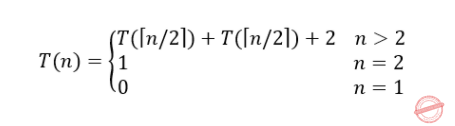When n is a power of two, n = 2k for some positive integer k, then

T(n)=2T(n/2)+2

=2(2T(n/4)+2)+2

=4T(n/4)+4+2

፧

=2k-1T(2)+1ik-12i

=2k-1+2k-2

=3n/2-2

→ The given example n=512

Apply into 3n/2 -2

= (3*512)/2 -2

= 768-2

= 766

Method-2:

Find the minimum and maximum independently, using n-1 comparisons for each, for a total of 2n-2 comparisons. In fact, at most 3⌊n/2⌋ comparisons are sufficient to find both the minimum and the maximum. The strategy is to maintain the minimum and maximum elements seen thus far. Rather than processing each element of the input by comparing it against the current minimum and maximum, at a cost of 2 comparisons per element, we process elements in pairs. We compare pairs of elements from the input first with each other, and then we compare the smaller to the current minimum and the larger to the current maximum, at a cost of 3 comparisons for every 2 elements.

Setting up initial values for the current minimum and maximum depends on whether n is odd or even. If n is odd, we set both the minimum and maximum to the value of the first element,and then we process the rest of the elements in pairs. If n is even, we perform 1 comparison on the first 2 elements to determine the initial values of the minimum and maximum, and then process the rest of the elements in pairs as in the case for odd n.

Let us analyze the total number of comparisons. If n is odd, then we perform 3⌊n/2⌋ comparisons. If n is even, we perform 1 initial comparison followed by 3(n-2)/2 comparisons, for a total of (3n/2)-2.  Thus, in either case, the total number of comparisons is at most 3⌊n/2⌋.

Given an even number of elements. So, 3n/2 -2 comparisons.

= (3*512)/2 -2

= 768-2

= 766

Method-3:

By using Tournament Method:

Step-1: To find the minimum element in the array will take n-1 comparisons.

We are given 512 elements. So, to find the minimum element in the array will take 512-1= 511

Step-2: To find the largest element in the array using the tournament method.

1. After the first round of Tournament , there will be exactly n/2 numbers =256 that will lose the round.
2. The biggest loser (the largest number) should be among these 256 loosers.To find the largest number will take (n/2)−1 comparisons =256-1 = 255

Total 511+255= 766

 Question 65
A binary search tree T contains n distinct elements. What is the time complexity of picking an element in T that is smaller than the maximum element in T?
 AB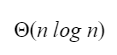CDData-Structures       Binary-search-tree
Question 65 Explanation:

The time complexity of searching an element in T that is smaller than the maximum element in T is O(1) time.

Example:Comparing that 5<10 will take only a constant amount of time.

There are 65 questions to complete.